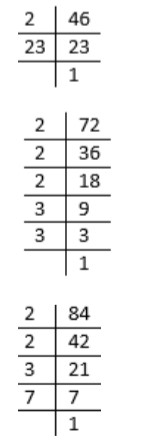QuestionAnswers

# Find the LCM of 46, 72, and 84 by prime factor method.

Hint: Find the prime factors of 46, 72, and 84. Multiply the highest powers of each prime factor. The product thus obtained is the LCM.

Complete step by step solution:
We are given three numbers 46, 72, and 84.
We are asked to compute their LCM. LCM stands for least common multiple
And the method to be used is the prime factor method.
In this method we need to prime factorize each of the given numbers.
Then we multiply all the prime factors with the highest power.
Consider the prime factorizations of 46, 72, and 84.Using the above computations, we will express the given numbers as products of their prime factors.
$46 = 2 \times 23 \\ 72 = 2 \times 2 \times 2 \times 3 \times 3 = {2^3} \times {3^2} \\ 84 = 2 \times 2 \times 3 \times 7 = {2^2} \times 3 \times 7 \\$
The prime factors are 2, 3, 7, and 23.
Highest power of 2$= {2^3}$
Highest power of 3$= {3^2}$
Highest power of 7$= 7$
Highest power of 23$= 23$
Therefore, LCM of 46, 72, and 84
$= {2^3} \times {3^2} \times 7 \times 23 \\ = 11,592 \\$
Hence the required LCM is 11,592.

Note: As the name suggests, while using the prime factor method, the number must be expressed as a product of its prime factors.
This is the difference between the prime factor method for LCM and the conventional method for finding LCM where we take into consideration the multiples.